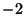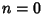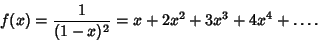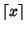## Integer

One of the numbers ...,,, 0, 1, 2, .... The Set of Integers forms a Ring which is denoted. A given Integermay be Negative (), Nonnegative (), Zero (), or Positive (). The Ringhas Cardinality of Aleph-0. The Generating Function for the Nonnegative Integers isThere are several symbols used to perform operations having to do with conversion between Real Numbers and integers. The symbol(Floor'') means the largest integer not greater than,'' i.e., int(x) in computer parlance. The symbolmeans the nearest integer to'' (Nint), i.e., nint(x) in computer parlance. The symbol(Ceiling'') means the smallest integer not smaller,'' or -int(-x), where int(x) is the Integer Part of.

See also Algebraic Integer, Almost Integer, Complex Number, Counting Number, Cyclotomic Integer, Eisenstein Integer, Gaussian Integer, N, Natural Number, Negative, Positive, Radical Integer, Real Number, Whole Number, Z, Z-, Z+, Z*, Zero